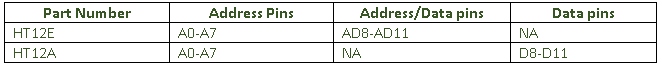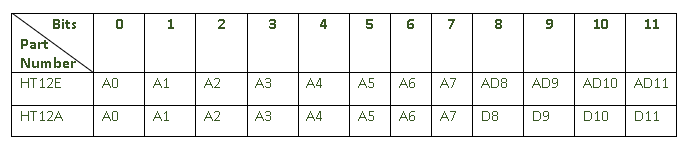top of page
Search

# Explained: Address and Data pins in HT12A/HT12E Encoder and HT12D/HT12F Decoder pair

Hello everyone! In this blog we will see the usage of address pins in HT12x encoder/decoder. To understand the purpose of address pins, it is necessary to understand the functionality of encoder and decoder and details about the available address and data pins.

Pin Diagram:Fig - 1 EncoderFig - 2 Decoder

Below table describes the Address and Data pins in Encoder and Decoder.

EncoderDecoderHow Encoder and Decoder works?

The encoder processes the input data and outputs 12-bits of serial data. The data input given to pins AD8-AD11 (in HT12E) or D8-D11 (in HT12A) are processed as binary bits (1 for High, 0 for Low) and additionally added with 8 address bits which came from the address pins (A0-A7). The final output from the encoder will be in below format.Table -1 Encoder Bit Format

For instance, assume that data inputs are given to the encoder HT12E as shown in Fig-2. For this explanation, all address bits are considered as 0 (i.e grounded).Fig - 2 Example Setup

For this data and address pin setup output format will be as below.Table - 2 Output Data Format

Hence ‘000000000010’ will be the output and the same will be given to the decoder at the receiver end.

Below table shows the bit format for HT12x decoders.Table - 3 Decoder Bit Format

Let’s consider HT12D decoder for our explanation. The decoder receives the data transmitted by the encoder and interprets first 8-bit as address bits and remaining 4-bits as data bits. In case of HT12F, all 12-bits will be interpreted as address bits.

Now we understood how encoding and decoding works in HT12x and the bit format. From here we will move to our next section i.e., the use of address bits.

We have seen the address pins in the pin diagram. We have these address pins in both encoder and decoder. So, what purpose it serves?

To find the answer, let’s consider a scenario. You have two pairs of Encoder (HT12E) and Decoder (HT12D) for different purposes. You set the address bits to low in all the four. You initiated the transmission from one encoder and notes that the information is decoded by both the decoders whereas the original requirement is to transmit the information to only one targeted decoder. How to achieve this? Well, here is where address bit comes into action.

The main purpose of address bit is to ensure security. It will make sure that only the targeted decoder will be able to decode the information. This will be achieved by setting up the encoder-decoder with same address bits. Upon receiving the information from encoder, the decoder compares the received address bits with its own and start to process output only if the address bits match.

Encoder and Decoder pair needs to be chosen accordingly as per the address and data bits format to gain proper functionality. For example, if you are using HT12A encoder, then HT12F won’t be the good choice to pair with since it doesn’t have any data bits.